## For a particular pipe in a pipe-organ, it has been determined that the frequencies 576 Hz and 648 Hz are two adjacent natural frequencies. U

Question

For a particular pipe in a pipe-organ, it has been determined that the frequencies 576 Hz and 648 Hz are two adjacent natural frequencies. Using 343 m/s as the speed of sound in air, determine the following:
(a) Fundamental frequency for this pipe.
(b) Is the pipe is open at both ends or closed at one end?
(c) Length of the pipe.

in progress 0
5 months 2021-08-28T11:18:44+00:00 1 Answers 8 views 0

(a). The fundamental frequency for the pipe is 72.0 Hz.

(b). This pipe is open at both ends.

(c). The length of the pipe is 2.38 m.

Explanation:

Given that,

Frequency f₁= 576 Hz

Frequency f₂ = 648 Hz

Speed of sound = 343 m/s

We need to calculate the length of the pipe

Using formula of frequency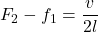Put the value into the formula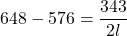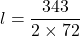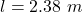(a).We need to calculate the fundamental frequency for the pipe

Using formula of fundamental frequency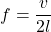Put the value into the formula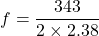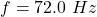This pipe is open at both ends.

Hence, (a). The fundamental frequency for the pipe is 72.0 Hz.

(b). This pipe is open at both ends.

(c). The length of the pipe is 2.38 m.